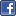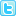# Abstract

## Prediction of the State of Cell Membrane Potassium Channel

In this paper, we have presented a method to predict the state of cell membrane Potassium channel in different voltages by using Markov model. One feature of models which are based on Markov is the demonstrating transition states so that prediction of ion channel behaviour will be possible. In this research, model parameters are obtained by Linearization of Macroscopic current, and then the probability of transfer from one state to another is calculated in terms of time and voltage. After that probability matrix of state changes of Markov model was found. By use of this matrix prediction of channel state in different voltages will be possible. In this article, the predicted channel state for 100 future states will be shown. Accuracy of this model is assessed by comparison between actual channel conductance that is obtained from macroscopic current of the software in different voltages and conductance that is obtained from the considered model. One of the most important advantages of this method of modelling is the capability of predicting the behaviour of ion channels in different voltages.

Author(s): Somayyeh Shahhosseini, Soheila Esmaeili P, S.H. Sabzposhan

Abstract | Full-Text | PDF

Share this###### Citations : 3582

Electronic Journal of Biology received 3582 citations as per google scholar report